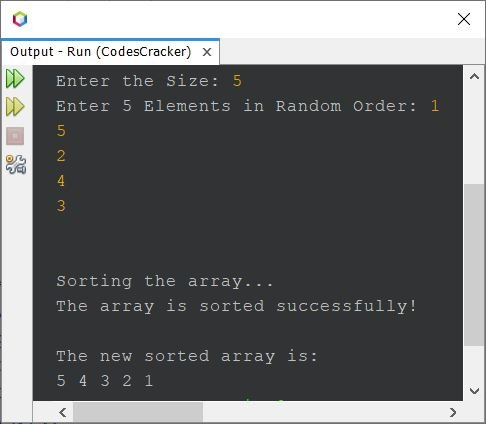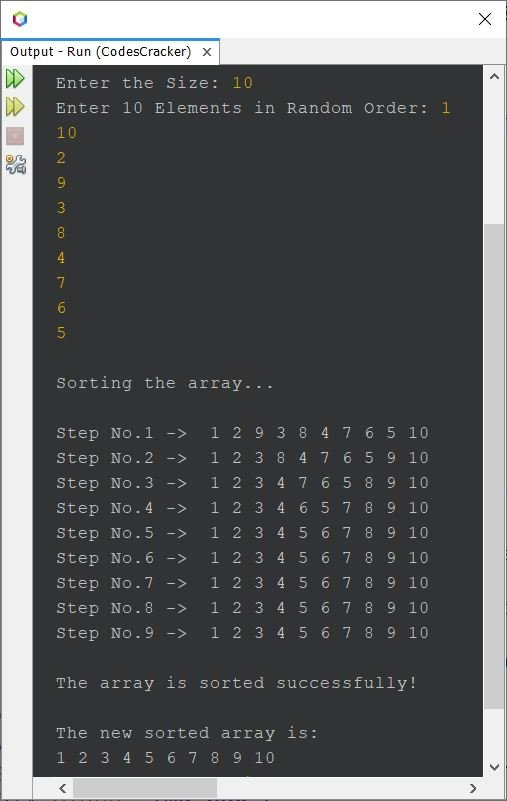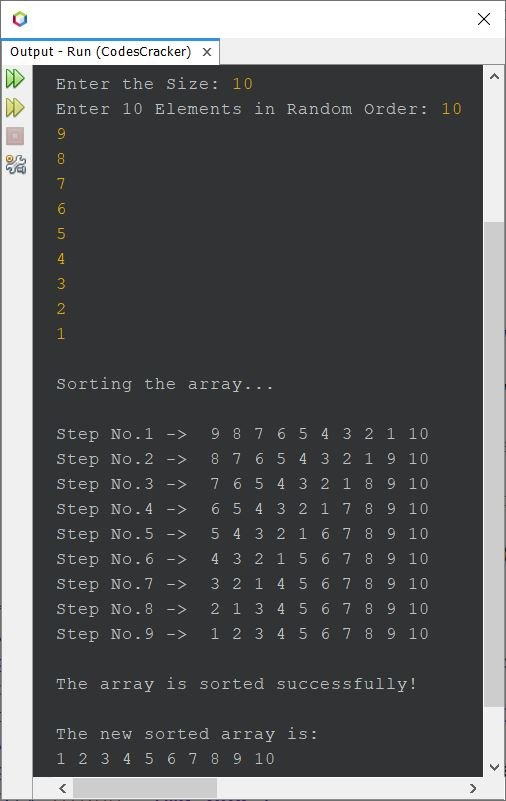# Java Program: Bubble Sort

• Bubble sort program in Java, basic version
• Bubble sort program in Java, complete version
• Bubble sort program in Java (descending order)
• Bubble sort with the printing of a new array after each sort

If you're not aware of it, How do bubble sorting works?
Refer to Bubble Sort Logic. Now let's move on and create a bubble sort program in Java.

## Bubble Sort in Java: Basic Version

The question is: write a Java program to perform bubble sort based on 10 elements. The 10 elements must be received by the user at runtime of the program. The program given below is its answer. This program sorts in ascending order.

```import java.util.Scanner;

public class CodesCracker
{
public static void main(String[] args)
{
int n=10, i, j, x;
int[] array = new int[n];
Scanner s = new Scanner(System.in);

System.out.print("Enter 10 Elements in Random Order: ");
for(i=0; i<n; i++)
{
array[i] = s.nextInt();
}

for(i=0; i<(n-1); i++)
{
for(j=0; j<(n-i-1); j++)
{
if(array[j]>array[j+1])
{
x = array[j];
array[j] = array[j+1];
array[j+1] = x;
}
}
}

System.out.println("\nThe new sorted array is:");
for(i=0; i<n; i++)
System.out.print(array[i]+ " ");
}
}```

The snapshot given below shows the sample run of the above bubble sort program in Java, with user input 10, 19, 11, 18, 12, 17, 13, 16, 14, and 15 as ten elements in random order. Random order means all the elements are neither in ascending nor descending order.## Bubble Sort in Java: Complete Version

Since the previous program operated based on only 10 elements or numbers, Therefore, I've modified that program to allow the user to define the size of the array along with its elements.

```import java.util.Scanner;

public class CodesCracker
{
public static void main(String[] args)
{
Scanner s = new Scanner(System.in);

System.out.print("Enter the Size: ");
int n = s.nextInt();

int[] arr = new int[n];

System.out.print("Enter " +n+" Elements in Random Order: ");
for(int i=0; i<n; i++)
arr[i] = s.nextInt();

System.out.println("\n\nSorting the array...");
for(int i=0; i<(n-1); i++)
{
for(int j=0; j<(n-i-1); j++)
{
if(arr[j]>arr[j+1])
{
int temp = arr[j];
arr[j] = arr[j+1];
arr[j+1] = temp;
}
}
}
System.out.println("The array is sorted successfully!");

System.out.println("\nThe new sorted array is:");
for(int i=0; i<n; i++)
System.out.print(arr[i]+ " ");
}
}```

The sample run with user input 5 as size and 1, 5, 2, 4, and 3 as five elements is shown in the snapshot given below:## Bubble Sort in Java: Descending Order

To sort an array in descending order using the bubble sort technique, only replace the greater than (>) sign with the less than (<), that is available inside the condition of the if statement in the sorting loop. That is, replace:

`if(arr[j]>arr[j+1])`

from the above program, with:

`if(arr[j]<arr[j+1])`

Only the matter of a sign, a single character. The whole program gets changed. Here is a snapshot of the sample run with the same user input as the previous program's sample run:## Bubble Sort in Java: Print an array after each sort

This is the last program in this article, created to print a new array after each sort using the bubble sort technique. This program provides a rough look at how the bubble sort actually performs.

```import java.util.Scanner;

public class CodesCracker
{
public static void main(String[] args)
{
Scanner s = new Scanner(System.in);

System.out.print("Enter the Size: ");
int n = s.nextInt();

int[] arr = new int[n];

System.out.print("Enter " +n+" Elements in Random Order: ");
for(int i=0; i<n; i++)
arr[i] = s.nextInt();

System.out.println("\nSorting the array...");
for(int i=0; i<(n-1); i++)
{
for(int j=0; j<(n-i-1); j++)
{
if(arr[j]>arr[j+1])
{
int temp = arr[j];
arr[j] = arr[j+1];
arr[j+1] = temp;
}
}
System.out.print("\nStep No." +(i+1)+ " ->  ");
for(int j=0; j<n; j++)
System.out.print(arr[j]+ " ");
}
System.out.println("\n\nThe array is sorted successfully!");

System.out.println("\nThe new sorted array is:");
for(int i=0; i<n; i++)
System.out.print(arr[i]+ " ");
}
}```

Here is its sample run with user input 10 as size and 1, 10, 2, 9, 3, 8, 4, 7, 6, and 5 as ten elements:Below is another sample run with the same user input as size and 10, 9, 8, 7, 6, 5, 4, 3, 2, 1 as ten elements:#### Same Program in Other Languages

Java Online Test

« Previous Program Next Program »